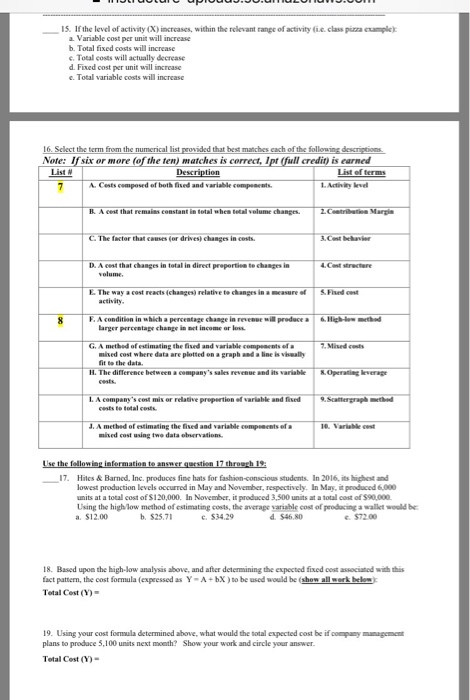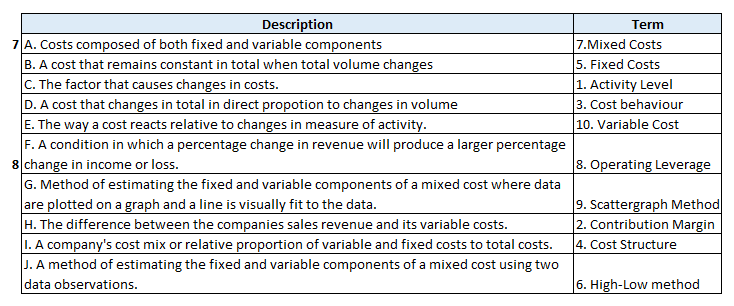# Question & Answer: If the level of activity(X) increases, within the relevant range of activity (i.e. class pizza example): a. Variable cost…..If the level of activity(X) increases, within the relevant range of activity (i.e. class pizza example): a. Variable cost per unit will increase b. Total fixed costs will increase c. Total costs will actually decrease d. Fixed cost per unit will increase e. Total variable costs will increase Select the term from the numerical list provided that best matches each of the following descriptions. Use the following information to answer question 17 through 19: Hites & Barned, Inc. produces fine hats for fashion-conscious students. In 2016, its highest and lowest production levels occurred in May and November, respectively. In May, is produced 6,000 units at a total cost of \$120, 000. In November, it produced 3,500 units at a total cost of \$90,000. Using the high/low method of estimating costs, the average variable cost of producing a wallet would be: a. \$12.00 b. \$25.71 c. \$34.29 d. \$46.80 c. \$72.00 Based upon the high-low analysis above, and after determining the expected fixed cost associated with this fact pattern, the cost formula (expressed as Y = A + bX) to be used would be (show all work below): Total Cost(Y) = Using your cost formula determined above, what would the total expected cost be if company management plans to produce 5,100 units next month? Show your work and circle your answer. Total Cost (Y) =